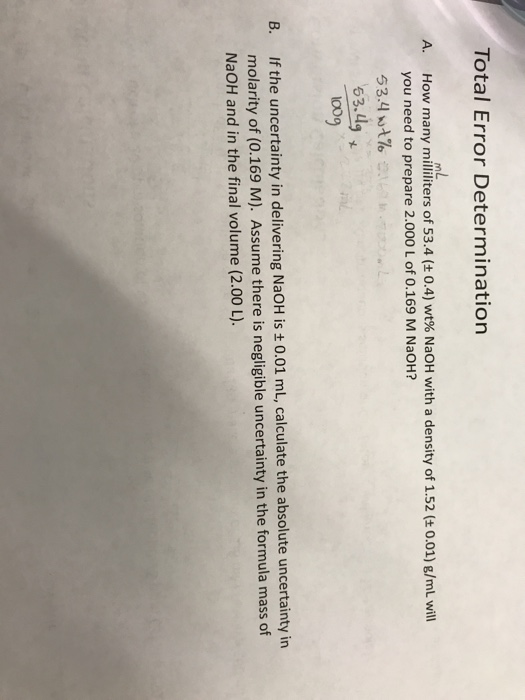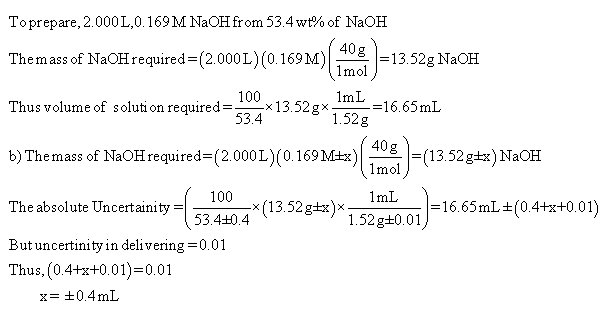# Question & Answer: How many milliliters of 53.4 (plusminus 0.4) wt% NaOH with a density of 1.52 (plusminus…..How many milliliters of 53.4 (plusminus 0.4) wt% NaOH with a density of 1.52 (plusminus 0.01) g/mL will you need to prepare 2.000 L of 0.169 M NaOH? If the uncertainty in delivering NaOH is plusminus 0.01 mL, calculate the absolute uncertainty in molarity of (0.169 M). Assume there is negligible uncertainty in the formula mass of NaOH and in the final volume (2.00 L).